# One Sample Score Test of a Proportion

Where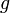$g$ is the observed proportion,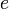$e$ is the expected proportion,$n$ is the effective sample size, and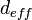$d_{eff}$ is Extra deff:$z = \frac{g - e}{\sqrt{d_{eff}e(1-e)/n}}$
where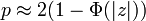$p \approx 2(1-\Phi(|z|))$ if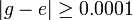$|g - e| \ge 0.0001$ and NaN otherwise.